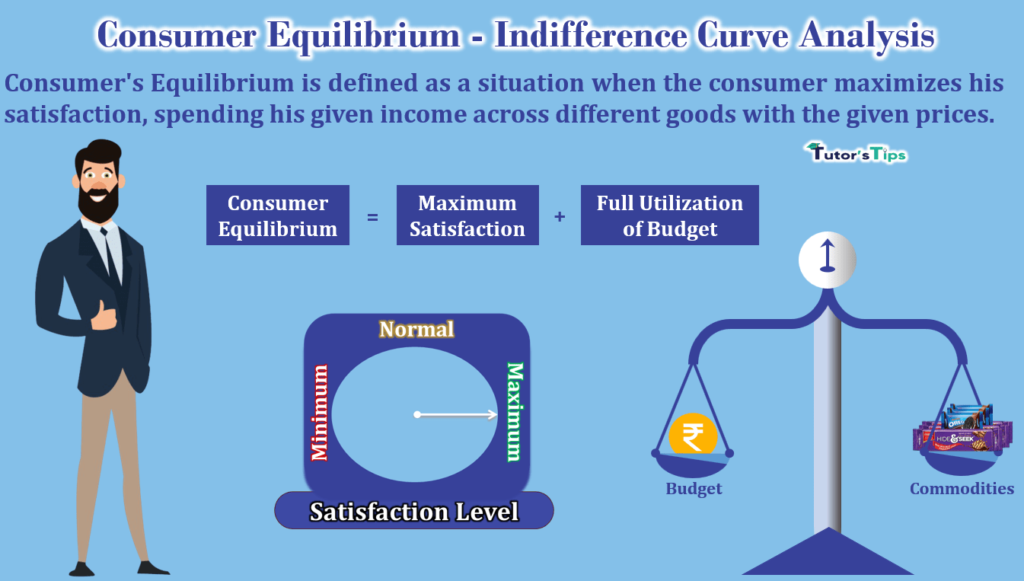# Consumer’s Equilibrium – Indifference Curve AnalysisThe consumer equilibrium through indifference curve analysis is based on the ordinal concept of utility. Here, the indifference Curve analysis measures where and how the consumer is getting maximum satisfaction with limited income by consuming two commodities.

## What is Consumer Equilibrium?

Consumer’s Equilibrium in Indifference Curve Analysis is defined as a situation when the consumer maximizes his satisfaction, spending his given income across different goods with the given prices. Here, the indifference curve and budget line are used to determine the consumer equilibrium point. Indifference curve analysis helps to find out how the consumer spends his limited income on the combination of different goods to get maximum satisfaction.

In other words, consumer’s equilibrium refers to a situation in which a consumer with given income and given prices purchases a combination of goods and services which gives him maximum satisfaction and he is not willing to make any change in it.

## Assumptions :

1. Money income of the consumer is given and is constant.
2. The two goods, on which income is spent, are a substitute for each other.
3. The consumer is rational and always tries to maximize his satisfaction.
4. Prices of goods are constant.
5. The consumer is aware of the prices prevailing in the market for all goods.
6. He can spend his income in small quantities.
7. There is perfect competition in the market.
8. The commodities are divisible.
9. The consumer is fully aware of the indifference map.

## Conditions of Consumer Equilibrium:

The consumer’s equilibrium under indifference curve analysis is found at the tangent between the budget line and a convex indifference curve. To find out the consumer equilibrium, the following conditions must be satisfied :

1. Price or Budget line should be tangent to an Indifference curve.
2. Indifference curve must be convex to the origin.

### 1. Price or Budget line should be tangent to an indifference curve:

In the words of Watson,

“When the consumer is in equilibrium, his highest attainable indifference curve is tangent to price line.”

In fig, AB is the budget or price line and IC1, IC2 and IC3 are indifference curves. A consumer can buy any of the combinations whether C, D and E  of chocolates Kitkat and DairyMilk, shown on budget line AB. He can’t buy any combination on ICas it is beyond the budget line AB. But, he can buy those combinations which are not only on the budget line AB but also coincide with the highest indifference curve which is IChere.

Out of the combinations C, D and E, the consumer will be at equilibrium at the combination D. Because, at this point, the budget line AB is tangent to the highest indifference curve IC2. No doubt, he can afford the combinations C and E as well but these will not give him the maximum satisfaction as these combinations belong to the lower indifference curve IC1.

It means, that the consumer’s equilibrium point is the point of tangency of the budget line and indifference curve. At point D, the slope of the indifference curve and budget line coincides. Here,

The slope of the indifference curve is indicative of the marginal rate of substitution of commodity-1 for commodity-2 (MRSXY).

The slope of the budget line is indicative of the ratio of the price of commodity-1(P1) and the price of commodity -2(P2).

#### At equilibrium:

Slope of indifference curve= =  Slope of Budget Line

Or

 MRSXY = P1 P2

In short, the first condition of the consumer’s equilibrium is that the budget or price line should be tangent to the indifference curve. It means that the price ratio of commodity-1 and commodity-2 should be equal to the marginal rate of substitution of commodity-1 for commodity-2.

### 2. Indifference curve must be convex to the origin:

The other condition of equilibrium is that at the point of equilibrium, the indifference curve should be convex to the point of origin. It means, that the marginal rate of substitution of commodity-1 for commodity-2 should be diminishing.

If at the point of equilibrium, the indifference curve is concave and not convex to the origin, then it will not be a position of permanent equilibrium.

#### Graphical Representation:

In fig, AB is the price line and IC is the indifference curve. At point ‘E’, price line AB is tangent to an indifference curve. Thus, at point E, the marginal rate of substitution and price ratio of KitKat and Dairy Milk are equal. But, at point E,  the marginal rate of substitution is increasing instead of diminishing. Therefore, E is not a permanent equilibrium point. In other words, at point E,  the indifference curve IS concave to its point of origin ‘O’ and it violates the second condition of the equilibrium.

By moving to the right or left of point E, a consumer can reach a higher indifference curve. So, equilibrium will not be permanent at point E. Therefore, A consumer will be in permanent equilibrium when at the point of tangency of the indifference curve and price line, the indifference curve is convex to the point of origin. As in fig, the consumer is in equilibrium at point E1 on IC1 curve. At the point E1, price line AB is tangent to IC1 curve which is convex to the point of origin.